Test: Banker's Discount- 1

# Test: Banker's Discount- 1

Test Description

## 10 Questions MCQ Test Logical Reasoning (LR) and Data Interpretation (DI) | Test: Banker's Discount- 1

Test: Banker's Discount- 1 for SSC CGL 2023 is part of Logical Reasoning (LR) and Data Interpretation (DI) preparation. The Test: Banker's Discount- 1 questions and answers have been prepared according to the SSC CGL exam syllabus.The Test: Banker's Discount- 1 MCQs are made for SSC CGL 2023 Exam. Find important definitions, questions, notes, meanings, examples, exercises, MCQs and online tests for Test: Banker's Discount- 1 below.
Solutions of Test: Banker's Discount- 1 questions in English are available as part of our Logical Reasoning (LR) and Data Interpretation (DI) for SSC CGL & Test: Banker's Discount- 1 solutions in Hindi for Logical Reasoning (LR) and Data Interpretation (DI) course. Download more important topics, notes, lectures and mock test series for SSC CGL Exam by signing up for free. Attempt Test: Banker's Discount- 1 | 10 questions in 10 minutes | Mock test for SSC CGL preparation | Free important questions MCQ to study Logical Reasoning (LR) and Data Interpretation (DI) for SSC CGL Exam | Download free PDF with solutions
 1 Crore+ students have signed up on EduRev. Have you?
Test: Banker's Discount- 1 - Question 1

### Q. The banker's discount on a bill due 4 months hence at 15% is Rs. 420. What is the true discount?

Detailed Solution for Test: Banker's Discount- 1 - Question 1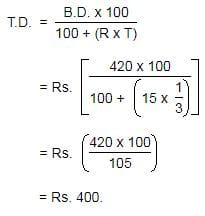Test: Banker's Discount- 1 - Question 2

### The banker's discount on a certain amount due 2 years hence is 11/10 of the true discount. What is the rate percent?

Detailed Solution for Test: Banker's Discount- 1 - Question 2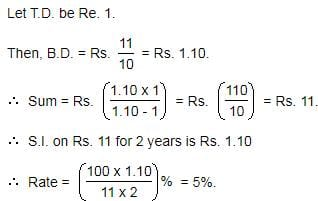Test: Banker's Discount- 1 - Question 3

### The present worth of a sum due sometime hence is Rs. 576 and the banker's gain is Rs. 16. The true discount is:

Detailed Solution for Test: Banker's Discount- 1 - Question 3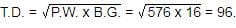Test: Banker's Discount- 1 - Question 4

What is the banker's discount if the true discount on a bill of Rs.540 is Rs.90 ?

Detailed Solution for Test: Banker's Discount- 1 - Question 4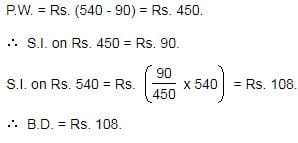Test: Banker's Discount- 1 - Question 5

A bill for Rs. 3000 is drawn on 14th July at 5 months. It is discounted on 5th October at 10%. What is the Banker's Discount?

Detailed Solution for Test: Banker's Discount- 1 - Question 5

F = Rs. 3000
R = 10%
Date on which the bill is drawn = 14th July at 5 months
Nominally Due Date = 14th December
Legally Due Date = 14th December + 3 days = 17th December
Date on which the bill is discounted = 5th October
Unexpired Time
= [6th to 31th of October] + [30 Days in November] + [1th to 17th of December]
= 26 + 30 + 17
= 73 Days
= 73/365year = 1/5year
Banker's Discount = Simple Interest on the face value of the bill for unexpired time = FTR/100
= (3000×1/5×10)/100
= 30×1/5×10
= Rs.60

Test: Banker's Discount- 1 - Question 6

The bankers discount and the true discount of a sum at 10% per annum simple interest for the same time are Rs.100 and Rs.80 respectively. What is the sum and the time?

Detailed Solution for Test: Banker's Discount- 1 - Question 6

BD = Rs.100
TD = Rs.80
R = 10%
F = BD×TD/(BD−TD)
= (100×80)/(100−80)
= (100×80)/20
= Rs.400
BD = Simple Interest on the face value of the bill for unexpired time = FTR/100
⇒ 100 = (400×T×10)/100
⇒ 100 = 4×T×10
⇒ 10 = 4×T
⇒ T = 10/4
= 2.5years

Test: Banker's Discount- 1 - Question 7

The banker's gain on a sum due 6 years hence at 12% per annum is Rs. 540. What is the banker's discount?

Detailed Solution for Test: Banker's Discount- 1 - Question 7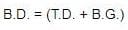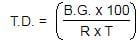Test: Banker's Discount- 1 - Question 8

The present worth of a certain bill due sometime hence is Rs. 1296 and the true discount is Rs. 72. What is the banker's discount?

Detailed Solution for Test: Banker's Discount- 1 - Question 8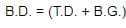and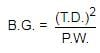Test: Banker's Discount- 1 - Question 9

The banker's discount of a certain sum of money is Rs. 36 and the true discount on the same sum for the same time is Rs. 30. What is the sum due?

Detailed Solution for Test: Banker's Discount- 1 - Question 9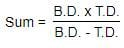Test: Banker's Discount- 1 - Question 10

The banker's gain on a bill due 1 year hence at 10% per annum is Rs. 20. What is the true discount?

Detailed Solution for Test: Banker's Discount- 1 - Question 10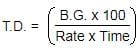## Logical Reasoning (LR) and Data Interpretation (DI)

96 videos|90 docs|119 tests
 Use Code STAYHOME200 and get INR 200 additional OFF Use Coupon Code
Information about Test: Banker's Discount- 1 Page
In this test you can find the Exam questions for Test: Banker's Discount- 1 solved & explained in the simplest way possible. Besides giving Questions and answers for Test: Banker's Discount- 1, EduRev gives you an ample number of Online tests for practice

## Logical Reasoning (LR) and Data Interpretation (DI)

96 videos|90 docs|119 tests